CAT  >  Practice Questions Level 1: Mixed Graphs

# Practice Questions Level 1: Mixed Graphs - Notes | Study Level-wise Practice Questions for CAT Preparation - CAT

 1 Crore+ students have signed up on EduRev. Have you?

This EduRev document offers 10 Multiple Choice Questions (MCQs) from the topic Mixed Graphs (Level - 1). These questions are of Level - 1 difficulty and will assist you in the preparation of CAT & other MBA exams. You can practice/attempt these CAT Multiple Choice Questions (MCQs) and check the explanations for a better understanding of the topic.

Question for Practice Questions Level 1: Mixed Graphs
Try yourself:Directions: Study the following stacked bar graph which represents the advertisement expenditure of a trading company from 1995 - 96 to 2000 - 01 on its products P, Q, R and S.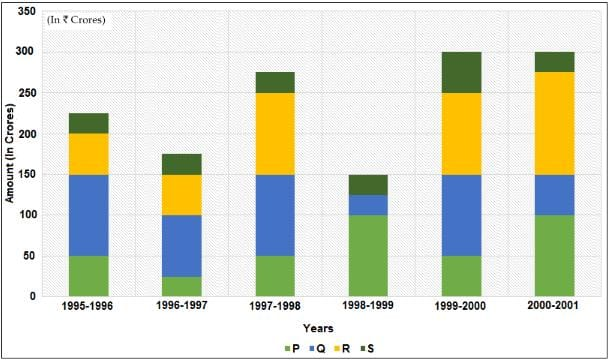Note: Sales for the given years are as follows: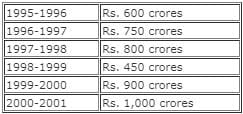Profit = Sales - Advertising Expenditure

Q. The advertising expenditure of product S as a percentage of the advertising expenditure of P and R together is least in which of the following years?

Question for Practice Questions Level 1: Mixed Graphs
Try yourself:Directions: Study the following stacked bar graph which represents the advertisement expenditure of a trading company from 1995 - 96 to 2000 - 01 on its products P, Q, R and S.Note: Sales for the given years are as follows:Profit = Sales - Advertising Expenditure

Q. If the ratio of sales of the products P, Q, R and S in the year 2000 - 01 is 2 : 5 : 1 : 2, then the profit of S is what percentage of the profit of Q in the same year?

Question for Practice Questions Level 1: Mixed Graphs
Try yourself:Directions: The given table gives information about the number of articles sold by store P, Q and R from August to November and the profit earned by each store by selling each article. Answer the question that follows.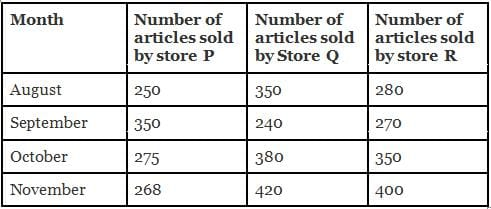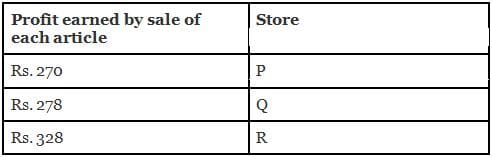Q. The total articles sold by stores P and Q from August to October is what percent more than total articles sold by store R from August to October?

Question for Practice Questions Level 1: Mixed Graphs
Try yourself:Directions: The given table gives information about the number of articles sold by store P, Q and R from August to November and the profit earned by each store by selling each article. Answer the question that follows.Q. What is the difference between the profit of all three stores in the month of September and the profit of stores Q and R in October from the sale of article?

Question for Practice Questions Level 1: Mixed Graphs
Try yourself:Directions: In the recently concluded UP Elections, a total of 2,50,000 e-votes were casted from 8 different cities mentioned below in the pie-chart. The table shows the ratio of e-votes received by BJP to SP.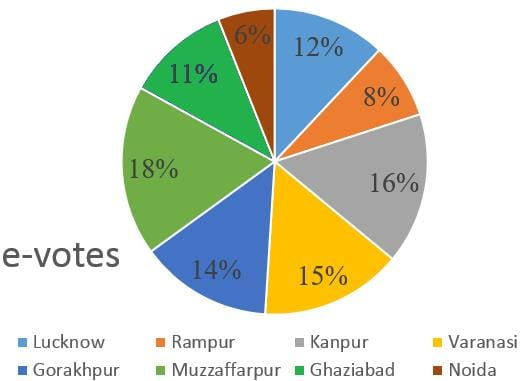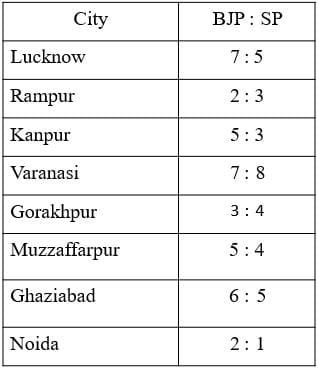Q. What is the ratio of e-votes received by BJP in Lucknow to those in Noida?

Question for Practice Questions Level 1: Mixed Graphs
Try yourself:Directions: In the recently concluded UP Elections, a total of 2,50,000 e-votes were casted from 8 different cities mentioned below in the pie-chart. The table shows the ratio of e-votes received by BJP to SP.Q. What is the ratio of the total number of e-votes cast to BJP in Kanpur and Varanasi to the total number of e-votes cast to SP in the same two cities?

Question for Practice Questions Level 1: Mixed Graphs
Try yourself:Directions: The pie chart given below shows the percentage population of seven different states in a particular year. The table shows sex and literacy ratios. Based on the given information, answer the following question: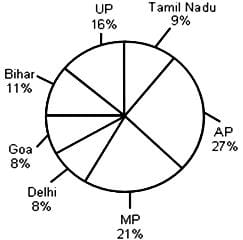Total population of the given states = 32,76,000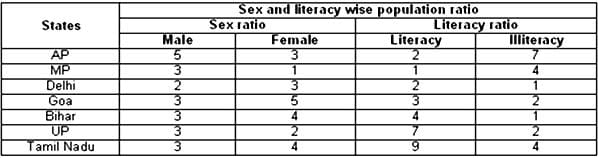Q. What is the ratio of the number of females in Tamil Nadu to that in Delhi?

Question for Practice Questions Level 1: Mixed Graphs
Try yourself:Directions: The pie chart given below shows the percentage population of seven different states in a particular year. The table shows sex and literacy ratios. Based on the given information, answer the following question:Total population of the given states = 32,76,000Q. What was the total number of illiterate people in AP and MP?

Question for Practice Questions Level 1: Mixed Graphs
Try yourself:Directions: Read the following information carefully to answer the question that follows.
Cost of different ingredients (in Rs./g) used in making food products: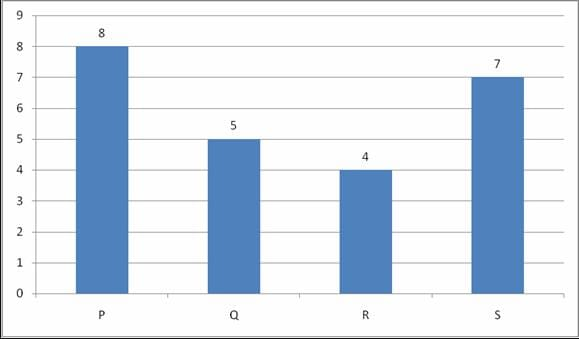Ratio of ingredients in each type of food products: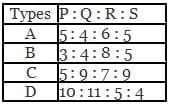Total cost of food products = Rs. 15 (making charges per food product) + ingredients cost

Q. What is the total cost of 500 g of type A and 600 g of type B food products?

Question for Practice Questions Level 1: Mixed Graphs
Try yourself:Directions: Read the following information carefully to answer the question that follows.
Cost of different ingredients (in Rs./g) used in making food products:Ratio of ingredients in each type of food products:Total cost of food products = Rs. 15 (making charges per food product) + ingredients cost

Q. What is the total cost of ingredients in 600 g food products of type B and 1200 g food of type C?

The document Practice Questions Level 1: Mixed Graphs - Notes | Study Level-wise Practice Questions for CAT Preparation - CAT is a part of the CAT Course Level-wise Practice Questions for CAT Preparation.
All you need of CAT at this link: CAT

## Level-wise Practice Questions for CAT Preparation

277 docs
 Use Code STAYHOME200 and get INR 200 additional OFF

## Level-wise Practice Questions for CAT Preparation

277 docs

### How to Prepare for CAT

Read our guide to prepare for CAT which is created by Toppers & the best Teachers

Track your progress, build streaks, highlight & save important lessons and more!

,

,

,

,

,

,

,

,

,

,

,

,

,

,

,

,

,

,

,

,

,

;